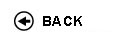Politecnico di Torino
Academic Year 2009/10
02BOWDR, 02BOWDS
Continuum Mechanics
1st degree and Bachelor-level of the Bologna process in Mechanical Engineering - Vercelli
Master of science-level of the Bologna process in Mechanical Engineering - Vercelli
 Teacher Status SSD Les Ex Lab Tut Years teaching Borri Brunetto MauroA2 ICAR/08 44 26 0 0 6
 SSD CFU Activities Area context ICAR/08 7 D - A scelta dello studente A scelta dello studente
 Objectives of the course The course presents the basis of the mechanics of elastic bodies starting from concepts like action, constraint, structural element, stress and strain. Equilibrium, compatibility and constitutive equations are presented for three-dimensional bodies and one-dimensional bodies. Statically indeterminate beam systems and the stability of equilibrium of slender rods are examined. Expected skills The student will be able to calculate the stress and the displacements produced in two-dimensional framed elastic structures by external forces. Prerequisites The contents of the following courses: Calculus I, Calculus II, Linear Algebra, Mechanics. Syllabus Kinematics and statics of rigid systems; calculation of reaction forces; internal reactions. Statically determinate beam systems: determination of internal and external reactions (graphical and analytical methods, virtual work theorem). Determination of internal forces (bending moment, normal and shear forces). Arches, Gerber beams, trussed and closed structures. Analysis of strain: strain tensor, stretch and rotation, transformation of strain tensor, principal directions, volumetric dilatation, compatibility equations. Analysis of stress: stress tensor, transformation of stress tensor, principal directions, hydrostatic and deviatoric tensor, Mohr's circle, plane stress, equilibrium equations, virtual work theorem. Elastic constitutive equations: linear elasticity, isotropy, homogeneity, elastic potential, elastic constants; theorems of Kirchhoff, Clapeyron and Betti. Saint-Venant's problem: hypotheses, centered axial loading, bending, eccentric axial loading; torsion (circular and general cross section), shear. Deflection of beams: second order differential equation. Solution of statically indeterminate structures: symmetry; force method, Müller-Breslau's method. Stability of elastic equilibrium of rods. Laboratories and/or exercises Exercises on many topics are presented and solved, aiming to enhance the understanding of the theory and to present the most important calculation techniques. Bibliography A. Carpinteri, Scienza delle Costruzioni, Pitagora Editrice, Bologna, vol. 1, 1995. A. Carpinteri, Scienza delle Costruzioni, Pitagora Editrice, Bologna, vol. 2, 1993. Revisions / Exam Final exam: a) multiple choice test; b) written test in two parts: problem solving and theoretical analysis; c) discussion of tests a and b. Programma definitivo per l'A.A.2009/10© Politecnico di Torino
Corso Duca degli Abruzzi, 24 - 10129 Torino, ITALY